# Samacheer Kalvi 12th Maths Solutions Chapter 5 Two Dimensional Analytical Geometry – II Ex 5.1

## Tamilnadu Samacheer Kalvi 12th Maths Solutions Chapter 5 Two Dimensional Analytical Geometry – II Ex 5.1

12th Maths Exercise 5.1 Question 1.
Obtain the equation of the circles with radius 5 cm and touching x-axis at the origin in general form.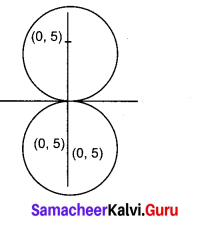Solution:
Given radius = 5 cm and the circle is touching x axis
So centre will be (0, ± 5) and radius = 5
The equation of the circle with centre (0, ± 5) and radius 5 units is
(x – 0)2 + (y ± 5)2 = 52
(i.e) x2 + y2 ± 10 y + 25 – 25 = 0
(i.e) x2 + y2 ± 10y = 0

12th Maths 5.1 Question 2.
Find the equation of the circle with centre (2, -1) and passing through the point (3, 6) in standard form.
Solution:
Centre = C = (2, -1); Passing through = A = (3, 6)
So radius = CA = $$\sqrt{(2-3)^{2}+(-1-6)^{2}}=\sqrt{1+49}=5 \sqrt{50}$$
Now centre = (2, -1) and radius = $$\sqrt{50}$$
So equation of the circle is
(i.e) (x – 2)2 + (y + 1)2 = $$\sqrt{50}^{2}$$ ⇒ (x – 2)2 + (y + 1)2 = 50

Two Dimensional Analytical Geometry 2 Question 3.
Find the equation of circles that touch both the axes and pass through (-4, – 2) in general form.
Solution:
Since the circle touches both the axes, its centre will be (r, r) and radius wil be r.
Here centre = C = (r, r) and point on the circle is A = (-4, -2)
CA = r ⇒ CA2 = r2
(i.e) (r + 4)2 + (r + 2)2 = r2
⇒ r2 + 8r +16 + r2 + 4r + 4 – r2 = 0
(i.e) r2 + 12r + 20 = 0
(r + 2) (r + 10) = 0
⇒ r = -2 or -10
When r = -2, the equation of the circle will be (x + 2)2 + (y + 2)2 = 22
(i.e) x2 + y2 + 4x + 4y + 4 = 0
When r = -10, the equation of the circle will be (x + 10)2 + (y + 10)2 = 102
(i.e) x2 + y2 + 20x + 20y + 100 = 0

Ex 5.1 Class 12 Question 4.
Find the equation of the circle with centre (2, 3) and passing through the intersection of the lines 3x – 2y -1 = 0 and 4x + y – 27 = 0 .
Solution:
To find the point of intersection of the two lines we have to solve the two equations.
Now solving them: 3x — 2y = 1 ……….. (1)
4x + y = 27 ……….(2)
(2) × 2 ⇒ 8x + 2y = 54 ……….. (3)
(1) ⇒ 3x – 2y = 1 ………. (1)
(3) + (1) ⇒ 11x = 55 ⇒ x = $$\frac{55}{11}$$ = 5
Substituting x = 5 in (2) we get
20 + y = 27 ⇒ y = 27 – 20 = 7
∴ The point = A = (5, 7)
Given centre = C = (2, 3)
∴ radius = $$\sqrt{(5-2)^{2}+(7-3)^{2}}=\sqrt{9+16}=5$$ = 5
Now centre = (2, 3) and radius = 5
So equation of the circle is
(x – 2)2 + (y – 3)2 = 52
(i.e) x2 + y2 – 4x – 6y – 12 =0

Chapter 5 Maths Class 12 Question 5.
Obtain the equation of the circle for which (3, 4) and (2, -7) are the ends of a diameter.
Solution:
The equation of a circle with (x1 , y1) and (x2 , y2 ) as end points of a diameter is
(x – x1 )(x – x2) + (y – y1 )(y – y2) = 0
Here the end points of a diameter are (3, 4) and (2, -7)
So equation of the circle is (x – 3 )(x – 2 ) + (y – 4) (y + 7.) = 0
x2 + y2 – 5x + 37 – 22 = 0

Exercise 5.1 Class 12 Question 6.
Find the equation of the circle through the points (1, 0), (-1, 0), and (0, 1).
Solution:
Let the required circle be
x2 + y2 + 2gx + 2fy + c = 0 …………. (A)
The circle passes through (1, 0), (-1, 0) and (0, 1)
(1, 0) ⇒ 1 + 0 + 2g(1) + 2f(0) + c = 0
2g + c = -1 ……………. (1)
(-1, 0) ⇒ 1 + 0 + 2g (-1) + 2f(0) + c = 0
-2g + c = -1 ……….. (2)
(0, 1) ⇒ 0 + 1 + 2g (0) + 2f(1) + c = 0
2f+ c = -1 ……….. (3)
Now solving (1), (2) and (3) .
2g + c = -1 ………… (1)
-2g + c = -1 ………….. (2)
(1) + (2) ⇒ 2c = -2 ⇒ c = -1
Substituting c = -1 in (1) we get
2g – 1 = -1
2g = -1 + 1 = 0 ⇒ g = 0
Substituting c = -1 in (3) we get
2f – 1 =-1 ⇒ 2f = -1 + 1 = 0 ⇒ f = 0
So we get g = 0, f= 0 and c = -1
So the required circle will be
x2 + y2 + 2(0) x + 2(0)y – 1 = 0
(i.e) x2 + y2 – 1 = 0 ⇒ x2 + y2 = 1

Two Dimensional Analytical Geometry Pdf Question 7.
A circle of area 9n square units has two of its diameters along the lines x + y = 5 and x – y = 1. Find the equation of the circle.
Solution:
Area of the circle = 9π
(i.e) πr2 = 9π
⇒ r2 = 9 ⇒ r = 3
(i.e) radius of the circle = r = 3
The two diameters are x + y = 5 and x – y = 1
The point of intersection of the diameter is the centre of the circle = C
To find C: Solving x + y = 5 ……… (1)
x – y = 1 ………. (2)
(1) + (2) ⇒ 2x = 6 ⇒ x = 3
Substituting x = 3 in (1) we get
3 + y = 5 ⇒ y = 5 – 3 = 2
∴ Centre = (3, 2) and radius = 3
So equation of the circle is (x – 3)2 + (y – 2)2 = 32
(i.e) x2 + y2 – 6x – 4y + 4 =0

Ex5 1 Class 12 Question 8.
If y = $$2 \sqrt{2} x$$ + c is a tangent to the circle x2 + y2 =16 , find the value of c .
Solution:
The condition for the line y = mx + c to be a tangent to the circle x2 + y2 = a2 is
c2 = a2(1 + m2)
Here x2 + y2 = 16 ⇒ a2 = 16
y = $$2 \sqrt{2} x$$ + c ⇒ m = $$2 \sqrt{2}$$ and c = c
The condition is c2 = a2 (1 + m2)
(i.e) c2 = 16(1 + 8) = 144
⇒ c = ± 12

Exercise 5.1 Maths Class 12 Question 9.
Find the equation of the tangent and normal to the circle x2 + y2 – 6x + 6y – 8 = 0 at (2, 2).
Solution:
The equation of the tangent to the circle x2 + y2 + 2 gx + 2fy + c = 0 at (x1, y1) is
xx1 + yy1 + g(x + x1) + f(y + y1) + c = 9
So the equation of the tangent to the circle
x2 + y2 – 6x + 6y – 8 = 0 at (x1, y1) is
xx1 + yy1 – $$\frac{6\left(x+x_{1}\right)}{2}+\frac{6\left(y+y_{1}\right)}{2}$$ – 8 = 0
(i.e) xx1 + yy1 – 3(x + x1) + 3(y + y1) – 8 = 0
Here (x1, y1) = (2, 2)
So equation of the tangent is
x(2) + y(2) – 3(x + 2) + 3(y + 2) – 8 = 0
(.i.e) 2x + 2y – 3x – 6 + 3y + 6 – 8 = 0
(i.e) -x + 5y – 8 = 0 or x – 5y + 8=0
Normal is a line ⊥r to the tangent
So equation of normal circle be of the form 5x + y + k = 0
The normal is drawn at (2, 2)
⇒ 10 + 2 + k = 0 ⇒ k = -12
So equation of normal is 5x + y – 12 = 0

Plus Two Maths Exercise 5.1 Question 10.
Determine whether the points (-2, 1), (0, 0) and (-4, -3) lie outside, on or inside the circle x2 + y2 – 5x + 2y – 5 = 0 .
Solution:
To find the position of a point with regard to a given circle, substitute the point in the equation of the circle if we get a positive value, the point lies outside the circle.
If we get a -ve value the point lies inside the circle and if we get O then the point lies on the circumference of the circle.
The given circle is x2 + y2 – 5x + 2y – 5 = 0 ……….. (1)
Substituting the point (-2, 1) in (1) we get
4 + 1 – 5(-2) + 2(1) – 5 = 5 + 10 + 2 – 5 = 12
⇒ (- 2, 1) lies outside the circle
Substituting the point (0, 0) in (1) we get
-5 < 0 ⇒ (0, 0) lies inside the circle Substituting the point (-4, -3) in (1) we get 16 + 9 + 20 – 6 – 5 = 34 >0
⇒ (- 4, -3) lies outside the circle

Exercise 5.1 Class 12 Maths Question 11.
Find centre and radius of the following circles.
(i) x2 + (y + 2)2 = 0
(ii) x2 + y2 + 6x – 4y + 4 = 0
(iii) x2 + y2 – x + 2y – 3 = 0
(iv) 2x2 + 2y2 – 6x + 4y + 2 = 0
Solution:
(i) x2 + (y + 2)2 = 0
(i.e) x2 + y2 + 4y + 4 = 0
Comparing this equation with the general form x2 + y2 + 2gx + 2fy + c = 0
we get 2g = 0 ⇒ g = 0
2f = 4 ⇒ f= 2 and c = 4
Now centre = (-g, -f) = (0, -2)
Radius = r = $$\sqrt{g^{2}+f^{2}-c}=\sqrt{0+4-4}$$
∴ Centre = (0, -2) and radius = 0

(ii) x2 + y2 + 6x – 4y + 4 = 0
Comparing with the general form we get
2g = 6, 2f = -4
⇒ g = 3, /= -2 and c = 4
Centre = (-g, -f) = (-3, 2)
Radius = $$\sqrt{g^{2}+f^{2}-c}=\sqrt{9+4-4}$$= 3
∴ Centre = (-3, 2) and radius = 3

(iii) x2 + y2 – x + 2y – 3 = 0
Comparing with the general form of the circle we get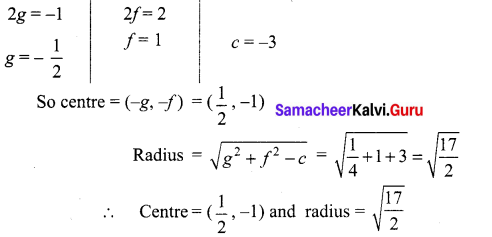(iv) 2x2 + 2y2 – 6x + 4y + 2 = 0
(÷ by 2) ⇒ x2 + y2 – 3x + 2y + 1 =0
Comparing this equation with the general form of the circle we get
2g = -3, 2f= 2
g = $$-\frac{3}{2}$$, g= 1 and c = 1
So centre = (-g, -f) = ($$\frac{3}{2}$$, -1)
and radius = $$\sqrt{g^{2}+f^{2}-c}=\sqrt{\frac{9}{4}+1-1}=\frac{3}{2}$$
∴ Centre = ($$\frac{3}{2}$$, -1) and radius = $$\frac{3}{2}$$

Class 12 Maths Ex5.1 Question 12.
If the equation 3x2 + (3 -p) xy + qy2 – 2px = 8 pq represents a circle, find p and q. Also determine the centre and radius of the circle.
Solution:
For a circle co-eff of x2 = co-efif of y2
⇒ 3 = q
co-eff of xy = 0
⇒ 3 – p = 0 ⇒ p = 3
So p = q = 3
So the equation of the circle becomes 3x2 + 3y2 – 6x – 72 = 0
(÷ by 3) ⇒ x2 + y2 – 2x – 24 = 0
Comparing this equation with the general form of the circle we get
2g = -2, 2f = 0
g = -1, f = 0 and c = -24
So centre = (-g, -f) = (1, 0) and radius = $$\sqrt{g^{2}+f^{2}-c}$$ = 5
∴ Centre =(1,0) and radius = 5

### Samacheer Kalvi 12th Maths Solutions Chapter 5 Two Dimensional Analytical Geometry – II Ex 5.1 Additional Problems

Exercise 5.1 Class 12 Solutions Question 1.
Find the equation of the circle whose centre is (2, -3) and passing through the intersection of the line 3x – 2y = 1 and 4x + y = 27.
Solution:
Solving 3x – 2y = 1 and 4x+y = 27
Simultaneously, we get x = 5 and y = 7
∴ The point of intersection of the lines is (5, 7)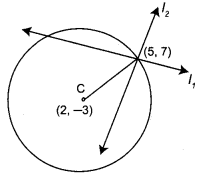Now we have to find the equation of a circle whose centre is (2, -3) and which passes through (5,7)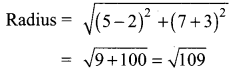∴ Required equation of the circle is
(x – 2)2 + (y + 3)2 = $$(\sqrt{109})^{2}$$
⇒ x2 + y2 – 4x + 6y – 96 = 0

Maths Class 12 Ex 5.1 Question 2.
Find the equation of a circle of radius 5 whose centre lies on x-axis and which passes through the point (2, 3).
Solution:
Let the centre of the circle be (h, k). Since the centre lies on x-axis, we have k = 0
Therefore, centre = (h, 0)
Also the circle has radius 5 units and passes through the point (2, 3).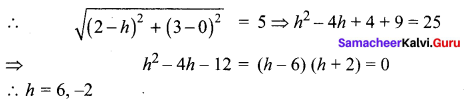Hence the centre is (6, 0) or (-2, 0)
∴ The equation of the circle is
(x – 6)2 + (y – 0)2 = 52 ⇒ x2 + y2 – 12x+ 11 = 0 (Or) (x + 2)2 + (y – 0)2 = 52 ⇒ x2 + y2 + 4x – 21 =0

Math Solution Class 12 Chapter 5 Question 3.
Find the centre and radius of the following circles:
x2 + y2 – 2x + 4y – 4 = 0 and 2x2 + 2y2 + 16x – 28y + 32 = 0. Also find the ratio of their diameters.
Solution:
Comparing the equation x2 + y2 – 2x + 4y – 4 = 0 with x2 + y2 + 2gx + 2 fy + c = 0, we get
2g = -2 ⇒ g = -1, 2f = 4 ⇒ f = 2, c = -4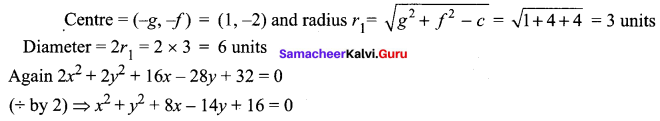Comparing with x2 + y2 + 2gx + 2fy + c = 0
2g = 8 ⇒ g = 4, 2f = -14 ⇒ f = -7, c = 16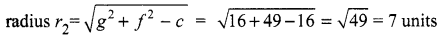Diameter = 2r2 = 2 × 7 = 14 units
Ratio of their diameters = 6 : 14 = 3 : 7.

Class 12 Math Chapter 5 Question 4.
Find the equation of the circle whose radius is 4 and which is concentric with the circle x2 + y2 + 2x – 6y = 0
Solution:
x2 + y2 + 2x – 6y = 0 …(1)
Here 2g = 2 ⇒ g = 1, 2f = -6 ⇒ f = -3
Centre of the circle = (-g, -f) = (-1, 3)
Since the required circle is concentric with (1), its centre is also (-1, 3).
∴ The equation of the circle whose centre is (-1, 3) and radius 4 is
(x + 1)2 + (y – 3)2 = 42
⇒ x2 + y2 + 2x – 6y – 6 = 0

Ex 5.1 Class 12 Maths Solutions Question 5.
Show that the four points (1, 0), (2, -7), (8,1) and (9, 6) are concyclic.
Solution:
Let the equation of the circle be Since it passes through (1,0) ⇒ x2 + y2 + 2gx + 2fy + c = 0 …(1)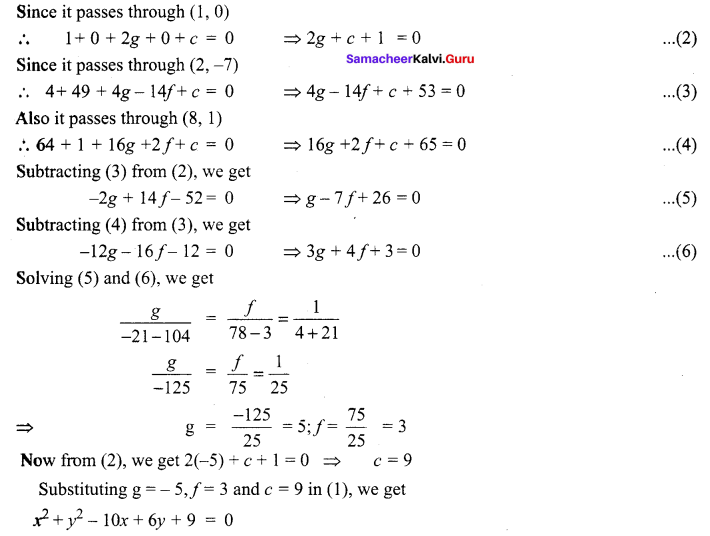Ex 5.1 Class 12 Pdf Question 6.
Find the equation of a circle which passes through the points (1, -2) and (4, -3) and whose centre lies on the line 3x + 4y = 0
Solution:
Let the equation of the circle be
x2 + y2 + 2gx + 2fy + c = 0 …(1)
Since it passes through (1, -2) and (4, -3)
∴ 5 + 2g – 4f + c = 0 …(2)
and 25 + 8g – 6f + c = 0 …(3)
Also (-g, -f) centre of circle (1) lies on 3x + 4y = 7
-3g – 4f = 7 …(4)
Subtracting (2) from (3), we get
20 + 6g – 2f = 0 …(5)
Solving (4) and (5), we get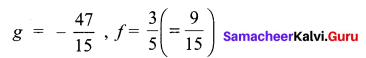Substituting these values of g and f in (2)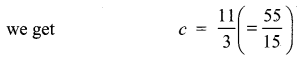∴ From (1), we get,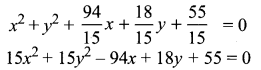Which is the required equation on the circle.

Question 7.
Find the equation (s) of the circle passing through the points (1, 1) and (2, 2) and whose radius is 1.
Solution:
Let the equation of the circle be
x2 + y2 + 2gx + 2fy + c = 0 …. (1)
Since it passes through (1,1)
∴ 1 + 1 + 2g + 2f + c = 0
⇒ 2g + 2f + c + 2 = 0 ….(2)
Again it passes through (2, 2)
∴ 4 + 4+ 4g + 4/+ c = 0
⇒ 4g + 4f + c + 8 = 0 …(3)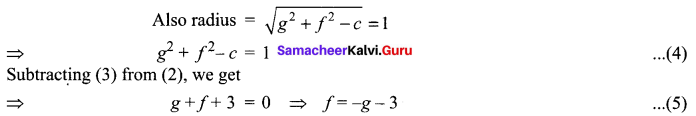g2 + 2g + f2 + 2f + 2 = 1
g2 + 2g + (-g – 3)2 + 2(-g – 3) + 1 = 0
⇒ 2g2 + 6g + 4 = 0 ⇒ g2 + 3g + 2 = 0
⇒ (g + 1)(g + 2) = 0 ⇒ g = -1, -2
when g = -1, from (5), f = – (-1) – 3 = 1 – 3 = -2
when g = -2, from (5), f = – (-2) – 3 = 2 – 3 = -1
Substituting g = -1, f = -2 in (2), we get
2(-1) + 2(-2) + c + 2 =0
⇒ – 6 + c + 2 = 0 ⇒ c = 4
Now putting g = -1, f = -2 and c = 4 in (1), we get
x2 + y2 – 2x – 4y + 4 = 0
Again putting g = -2, f = -1 in (2), we get
2(-2) + 2(-1) + c + 2 =0
⇒ -4 – 2 + c + 2 =0 ⇒ c = 4
Putting g = -2, f = -1 and c = 4 (in) (1), we get
x2 + y2 – 4x – 2y + 4 = 0
Hence the required equation (s) of the circle are
x2 + y2 – 2x – 4y + 4 =0 and x2 + y2 – 4x – 2y + 4 = 0.